# JEE Main 2020 Chemistry Paper With Solutions Jan 7 Shift 2

JEE Main 2020 solved shift 2 Chemistry paper consists of accurate solutions, which helps students to easily learn the problems. These are prepared by our subject experts. Practising these solutions will help students to self analyse their preparation level and thus find out their weaker areas and give more concentration to those topics. Students can easily download these solutions from our website in PDF format for free.

### January 7 Shift 2 - Chemistry

1. Consider the following reactions: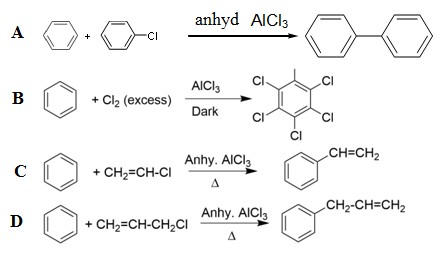Which of these reactions are possible?

1. a. A and D
2. b. B and D
3. c. B, C and D
4. d. A and B

Solution:

1. In aryl halides, due to the partial double bond character generated by chlorine, the aryl cation is not formed.

Vinyl halides do not give Friedel-Crafts reaction, because the intermediate that is generated (vinyl cation) is not stable.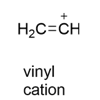2. In the following reaction sequence,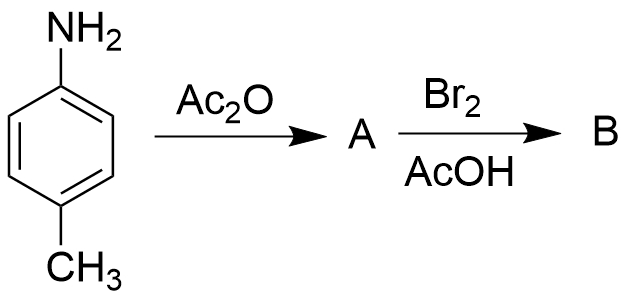The major product B is: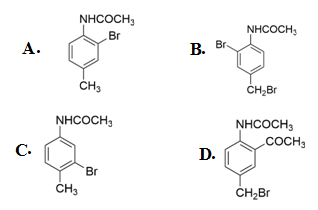Solution:

1. During trisubstitution, the acetanilide group attached to the benzene ring is more electron donating than the methyl group attached, owing to +M effect, and therefore, the incoming electrophile would prefer ortho w.r.t the acetanilide group.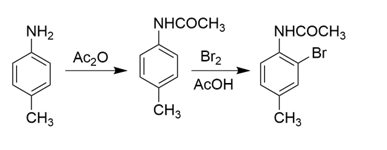3. For the following reactions,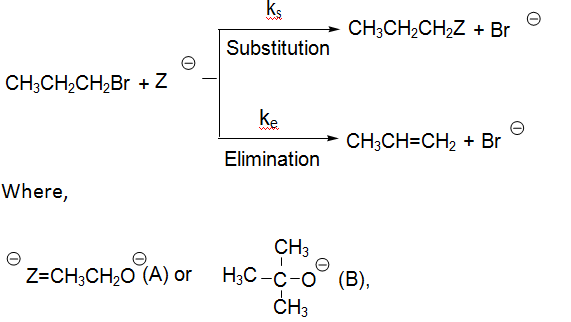ks and ke are respectively the rate constants for substitution and elimination, and μ = ks/ke, the correct option is

1. a. μA > μB and ke(A) > ke(B)
2. b. μB > μA and ke(A) > ke(B)
3. c. μA > μB and ke(B) > ke(A)
4. d. μB > μA and ke(B) > ke(A)

Solution:

1. The base CH3 CH20- favours substitution reaction over elimination reaction and thus 𝑘(A) <ks(𝐴).

Since the bulkier base tertiary butoxide (B) favours elimination reaction over substitution reaction, 𝑘 (B) is higher. Since μ = ks/ke , μA will be greater as it is having lower 𝑘𝑒 value.

4. Which of the following statements is correct?

1. a. Gluconic acid can form cyclic (acetal/hemiacetal) structure
2. b. Gluconic acid is a dicarboxylic acid
3. c. Gluconic acid is obtained by oxidation of glucose with HNO3
4. d. Gluconic acid is a partial oxidation product of glucose

Solution:

1. The gluconic acid formed is a monocarboxylic acid which is formed during the partial oxidation of glucose. Glucose on reaction with HNO3 will give glucaric acid: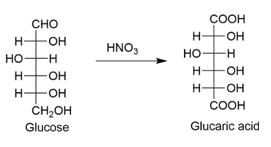Glucose on partial reduction will give gluconic acid.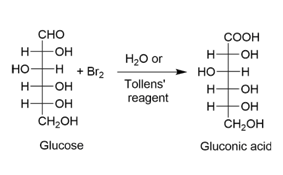5. The correct order of stability for the following alkoxides is: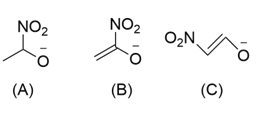1. a. (C) > (A) > (B)
2. b. (C) > (B) > (A)
3. c. (B) > (A) > (C)
4. d. (B) > (C) > (A)

Solution:

1. Higher the delocalization of the negative charge, more will be the stability of the anion.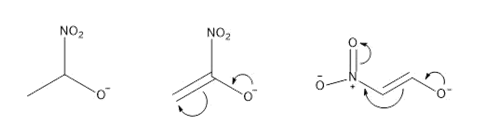a. The negative charge is stabilized only through –I effect exhibited by the – NO2 group.

b. The negative charge is stabilized by the delocalization of the double bond and the –I effect exhibited by the – NO2 group.

c. The negative charge is stabilized by extended conjugation.

6. In the following reaction sequence, structures of A and B, respectively will be: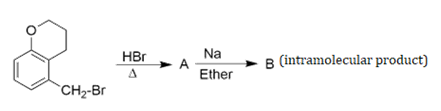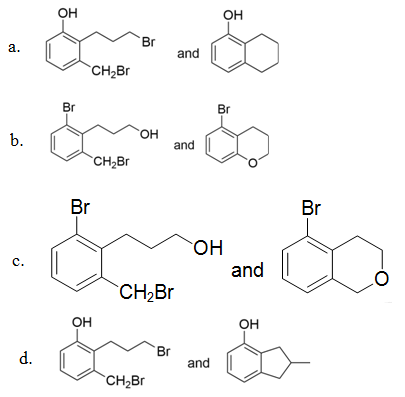Solution:

1.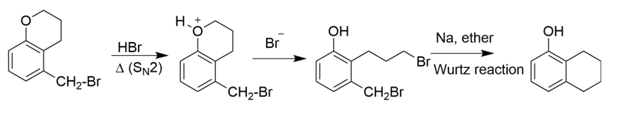7. A chromatography column, packed with silica gel as stationary phase, was used to separate a mixture of compounds consisting of (A) benzanilide, (B) aniline and (C) acetophenone. When the column is eluted with a mixture of solvents, hexane : ethyl acetate (20:80), the sequence of obtained compounds is:

1. a. (B), (A) and (C)
2. b. (C), (A) and (B)
3. c. (B), (C) and (A)
4. d. (A), (B) and (C)

Solution:

1. Since the column is eluted with the solvent having more proportion of ethyl acetate, the more polar compound will come out first.

The order of polarity in the given compounds is ; acetophenone > benzanilide > aniline.

(Dipolemoment (μ) of acetophenone = 3.05 D, benzanilide = 2.71 D and aniline = 1.59 D).

8. The number of possible optical isomers for the complexes [MA2 B2] with sp3 or dsp2 hybridized metal atom, respectively, is:

Note: A and B are unidentate neutral and unidentate monoanionic ligands, respectively.

1. a. 0 and 1
2. b. 0 and 0
3. c. 2 and 2
4. d. 0 and 2

Solution:

1. Case 1: If M is sp3 hybridized, the geometry will be tetrahedral. There will be a plane of symmetry and thus it does not show optical activity.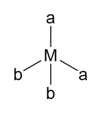Case 2: If M is dsp2 hybridized, the geometry will be square planar. Due to the presence of a plane of symmetry, it does not show optical activity.

9. The bond order and magnetic characteristics of CN- are:

1. a. 3, paramagnetic
2. b. 2.5, diamagnetic
3. c. 3, diamagnetic
4. d. 2.5, paramagnetic

Solution:

1. CN- is a 14 electron system. The bond order and magnetism can be predicted using MOT. The MOT electronic configuration of CN- is: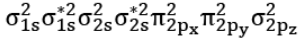Bond order = ½ ( Nbonding - Nantibonding) = 3

As CN- does not have any unpaired electrons, and hence it is diamagnetic.

10. The equation that is incorrect is

1. a. λ0mNaBr - λ0mNaI = λ0mKBr - λ0mNaBr
2. b. λ0mNaBr - λ0mNaCl = λ0mKBr - λ0mKCl
3. c. λ0mKCl - λ0mNaCl = λ0mKBr - λ0mNaBr
4. d. λ0mH2O = λ0mHCl + λ0mNaOH - λ0mNaBr

Solution:

1. λ0mNaI - λ0mNaBr = λ0mNaBr - λ0mKBr

0m Na+ + λ0m I-] - [λ0m Na+ + λ0m Br-] = [λ0m Na+ + λ0m Br-] - [λ0m K+0m Br-]

λ0m I- - λ0m Br- ≠ λ0m Na+ - λ0m K+

11. In the following reactions, product (A) and (B), respectively, are:

NaOH + Cl2 -> (A) + side products (hot & conc.)

Ca(OH)2+ Cl2 -> (B) + side products (dry)

1. a. NaClO3 and Ca(ClO3)2
2. b. NaOCl and Ca(ClO3)2
3. c. NaOCl and Ca(OCl)2
4. d. NaClO3 and Ca(OCl)2

Solution:

1. 6NaOH + 3Cl2 -> 5NaCl + NaClO3 + 3H2O

2Ca(OH)2 + Cl2 -> Ca(OCl)2 + CaCl2 + H2O

12. Two open beakers one containing a solvent and the other containing a mixture of that solvent with a non-volatile solute are together sealed in a container. Over time:

1. a. the volume of the solution and the solvent does not change
2. b. the volume of the solution increases and the volume of the solvent decreases
3. c. the volume of the solution decreases and the volume of the solvent increases
4. d. the volume of the solution does not change and the volume of the solvent decreases

Solution:

1. Consider beaker I contains the solvent and beaker 2 contains the solution. Let the vapour pressure of the beaker I be Po and the vapour pressure of beaker II be Ps . According to Raoult’s law, the vapour pressure of the solvent (Po ) is greater than the vapour pressure of the solution (Ps )

(Po > Ps)

Due to a higher vapour pressure, the solvent flows into the solution. So volume of beaker II would increase. In a closed beaker, both the liquids on attaining equilibrium with the vapour phase will end up having the same vapour pressure. Beaker II attains equilibrium at a lower vapour pressure and so in its case, condensation will occur so as to negate the increased vapour pressure from beaker I, which results in an increase in its volume.

On the contrary, since particles are condensing from the vapour phase in beaker II, the vapour pressure will decrease. Since beaker I at equilibrium attains a higher vapour pressure evaporation will be favoured more so as to compensate for the decreased vapour pressure, as mentioned in the previous statement.

13. The refining method used when the metal and the impurities have low and high melting temperatures, respectively, is:

1. a. vapour phase refining
2. b. distillation
3. c. liquation
4. d. zone refining

Solution:

1. Liquation is the process of refining a metal with a low melting point containing impurities of high melting point.

14. Among statements I-IV, the correct ones are:

I. Decomposition of hydrogen peroxide gives dioxygen

II. Like hydrogen peroxide, compounds, such as KClO3, Pb(NO3)2 and NaNO3 when heated liberate dioxygen.

III. 2-Ethylanthraquinone is useful for the industrial preparation of hydrogen peroxide.

IV. Hydrogen peroxide is used for the manufacture of sodium perborate

1. a. I,II, III and IV
2. b. I, II and III only
3. c. I, III and IV only
4. d. I and III only

Solution:

1. Decomposition of H2O2 : 2H2O2(l) → O2(g) + 2H2O(l)

Industrially, H2O2 is prepared by the auto-oxidation of 2-alklylanthraquinols.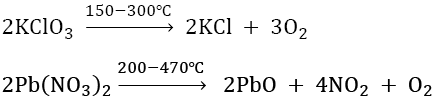2NaNO3 -> 2NaNO2 + O2

Synthesis of sodium perborate:

Na2B4O7 + 2NaOH + 4H2O2 -> 2NaBO3 + 5H2O

15. The redox reaction among the following is:

1. a. formation of ozone from atmospheric oxygen in the presence of sunlight
2. b. reaction of H2SO4 with NaOH
3. c. combination of dinitrogen with dioxygen at 2000 K
4. d. Reaction of [Co(H2O)6]Cl3 withAgNO3

Solution:

1. N2 + O2 (at 2000K) 2NO

The oxidation state of N changes from 0 to +2, and the oxidation state of O

changes from 0 to -2

In all the remaining reactions, there is no change in oxidation states of the elements participating in the reaction.

3O2 -> 2O3

2NaOH + H2SO4 -> Na2SO4 + 2H2O (Neutralisation reaction)

3 AgNO3 + [Co(H2O)6]Cl3 -> [Co(H2O)6](NO3)3 + 3 AgCl (Double displacement)

16. Identify the correct labels of A, B and C in the following graph from the options given below: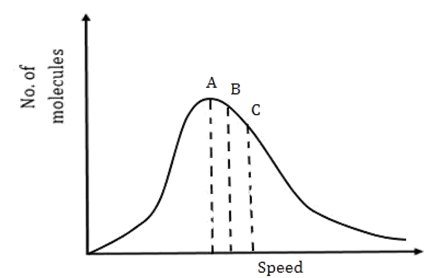Root mean square speed (Vrms); most probable speed (Vmps); average speed (Vav)

1. a. A = Vmps, B = Vav, C = Vrms
2. b. A = Vmps, B = Vrms, C = Vav
3. c. A = Vav, B = Vrms, C = Vmps
4. d. A = Vrms, B = Vmps, C = Vav

Solution:

1. CRMS = √(3RT/M)

CAverage = √(8RT/M)

CMPS = √(2RT/M)

√3 > √(8/) > √2

CRMS > CAverage > CMPS

17. For the reaction,

2H2(g) + 2NO(g) -> N2(g) + 2H2O(g)

The observed rate expression is, rate = kf[NO]2[H2]. The rate expression for the reverse reaction is:

1. a. kb[N2][H2O]2
2. b. kb[N2][H2O]
3. c. kb[N2][H2O]2/[H2]
4. d. kb[N2][H2O]2/[NO]

Solution:

1. The reverse reaction is:

2H2(g) + 2NO(g) ⇌ N2(g) + 2H2O(g)

The equilibrium constant,

Kc = kf/kb =

$$\frac{[N_{2}][H_{2}O]^{2}}{[H_{2}]^{2}[NO]^{2}}$$

At equilibrium, rate of forward reaction = rate of backward reaction

i.e., kf[NO]2[H2] = kb[N2][H2O]2/[H2]

Hence, rate of reverse reaction =

$$k_{b}\frac{[N_{2}][H_{2}O]^{2}}{[H_{2}]}$$

18. Within each pair of elements F & Cl, S & Se and Li & Na, respectively, the elements that release more energy upon an electron gain are:

1. a. Cl, Se and Na
2. b. Cl, S and Li
3. c. F, S and Li
4. d. F, Se and Na

Solution:

1.  Element First Electron gain enthalpy(kJ/mol) Li -60 Na -53 F -320 S -200 Cl -340 Se -195

Despite F being more electronegative than Cl, due to the small size of F, Cl would have a more negative value of electron gain enthalpy because of inter-electronic repulsions.

As we go down the group, the negative electron gain enthalpy decreases.

19. Among the following statements A-D, the incorrect ones are:

A. Octahedral Co(III) complexes with strong field ligands have high magnetic moments

B. When Δo < P, the d- electron configuration of Co(III) in an octahedral complex is t42g , e2g

C. Wavelength of light absorbed by [Co(en)3]3+is lower than that of [CoF6]3−.

D. If the Δ0 for an octahedral complex of Co(III) is 18000 cm−1, the Δt for its tetrahedral complex with the same ligand will be16000 cm−1 .

1. a. B and C only
2. b. A and B only
3. c. A and D only
4. d. C and D only

Solution:

1. Co3+ has d6 electronic configuration. In the presence of strong field ligand, Δo > P. Thus the splitting occurs as: t62g , e0g; so the magnetic moment is zero.

According to the spectrochemical series, en is a stronger ligand than F and therefore promotes pairing. This implies that the Δo of en is more than the Δo of F.

Δo = hc/abs

Δt = (4/9) Δ0

= 8000 cm-1

20. The ammonia (NH3) released on quantitative reaction of 0.6 g urea (NH2CONH2) with sodium hydroxide (NaOH) can be neutralized by:

1. a. 200 mL of 0.2 N HCl
2. b. 200 mL of 0.4 N HCl
3. c. 100 mL of 0.1 N HCl
4. d. 100 mL of 0.2 N HCl

Solution:

1. Moles of urea = 0.6/ 60 = 0.01

NH2CONH2+2NaOH -> Na2CO3+2NH3

0.02 moles of NH3 reacts with 0.02 moles of HCl.

Moles of HCl in option a = 0.2(100/1000) = 0.02

21. Number of sp2 hybrid carbon atoms present in aspartame is

Solution:

1.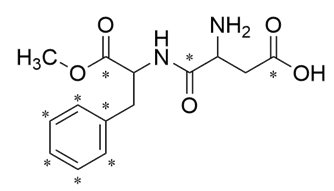The marked carbons are sp2 hybridised.

22. 3 grams of acetic acid is added to250 mL of 0.1 M HCl and the solution is made up to 500 mL. To

20 mL of this solution ½ mL of 5 M NaOH is added. The pH of this solution is:

(Given: log 3 = 0.4771, pKa of acetic acid = 4.74, molar mass of acetic acid = 60 g/mole).

Solution:

1. mmole of acetic acid in 20 mL = 2

mmole of HCl in 20 mL = 1

mmole of NaOH = 2.5

HCl + NaOH → NaCl + H2O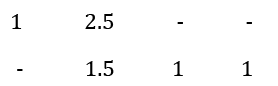CH3COOH + NaOH (remaining) -> CH3COONa + water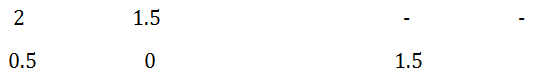pH = pKa + log (1.5/0.5) = 4.74 + log 3 = 4.74 + 0.48 = 5.22

23. The flocculation value of HCl for As2S3 sol is 30 m mol L–1. If H2SO4 is used for the flocculation of arsenic sulphide, the amount, in grams, of H2SO4 in 250 mL required for the above purpose is

Solution:

1. For 1L sol 30 mmol of HCl is required.

For 1L sol 15 mmol of H2SO4 is required .

For 250 mL of sol, (15/4)×98 ×10-3 g of H2SO4 = 0.3675 g

24. Consider the following reactions :

NaCl+K2Cr2O7+ H2SO4 -> (A)+side products

(A)+NaOH ->(B)+side products

(B)+H2SO4(dil.)+H2O2 ->(C)+side products

The sum of the total number of atoms in one molecule of (A),(B) & (C) is

Solution:

1.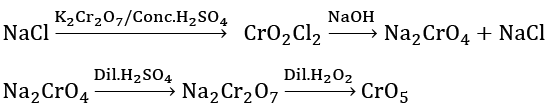(A) = CrO2Cl2,(B) = Na2CrO4 and (C) = CrO5

25. The standard heat of formation (ΔfH298°) of ethane (in kJ/mol), if the heat of combustion of ethane, hydrogen and graphite are -1560, -393.5 and -286 kJ/mol, respectively, is.

Solution:

1. C(graphite) + O2(g) → CO2(g)

ΔHc°=−286 kJ/mol…………………….(1)

H2(g)+ 0.5O2(g)→H2O(l)

ΔHc°= −393.5 kJ/mol ………………(2)

C2H6(g) + 3.5O2(g) → 2CO2(g) + 3H2O(l)

ΔHc°= −1560 kJ/mol …. (3)

2C(graphite)+3H2(g)→ C2𝐻6(g) ;

ΔH𝑓°= ?

By inverting (3) and multiplying (1) by 2 and (2) by 3 and adding, we get,

2 × (–286) + 3 × (–393.5) – (–1560) = –192.5 kJ/mol

### JEE Main 2020 Chemistry Paper January 7 Shift 2